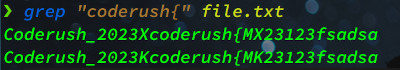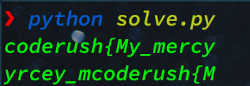# Coderush-1 Regex Showdown CTF Writeup

Contents

This challange requires observation to find a pattern in the text and apply a regex to find all instances of that pattern and construct the flag

The flag is spread throughout the file. There are lines which are in this format : patternXpattern where X is partial content of the flag. The flag can be constructed by concatenating all such X in the entire file (you dont need to reorder)

If we look into the file we can see that the whole flag might be there as there are “{” and “}” characters present.

We can search for “{” in the file but out first instinct should be searching for “coderush{”We can guess the pattern might be, Coderush2023X(.)X or Coderush2023K(.)K

Now lets write a python script to find out what these regex will give us,

 `````` 1 2 3 4 5 6 7 8 9 10 `````` ``````import re f = open("file.txt", "r") txt = f.read() match1 = re.findall(r'Coderush_2023X(.*)X', txt) match2 = re.findall(r'Coderush_2023K(.*)K', txt) print("".join(match1)) print("".join(match2)) ``````

The result is,So we are getting somewhere with the first pattern, but its not enough

Lets search for “}” now,Previously we have seen that “coderush{” is contained inside Coderush_2023X(.*)X So we should search for patterns which is a superset of this one

Lets analyze the relevant lines, we will change the pattern in the regex of our python script and see if that gives us the flag

linedeductioncombined patternresult
Coderush_2013X{DUMMY_FLAG}Y2132DSA90…X(.*)Y - doesn’t match with initial pattern
Coderush.9013Xflag}XPPDJ92103321There is . instead _ and instead of 2023 there are other digits but it can be a supersetCoderush[_.]\d{4}X(.*)Xcoderush{My_mercybraces_allflag}_7:1
 ``````1 `````` ``````8:CoderushUnderscore|not-4n{start_of_flagX2103XSD ``````
 ``````1 2 3 4 5 6 7 8 `````` ``````import re f = open("file.txt", "r") txt = f.read() match = re.findall(r'Coderush_?\d{4}X(.*)X', txt) print("".join(match)) ``````